# Welcome to Altadena Ms Sunday Key Points for

• Slides: 7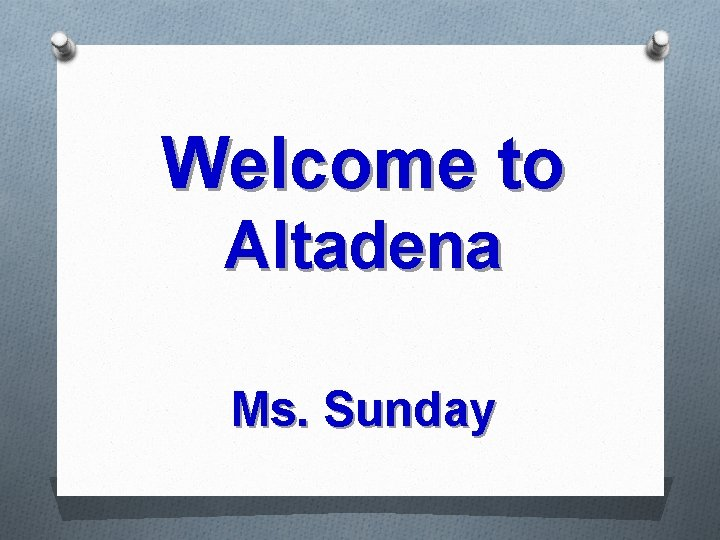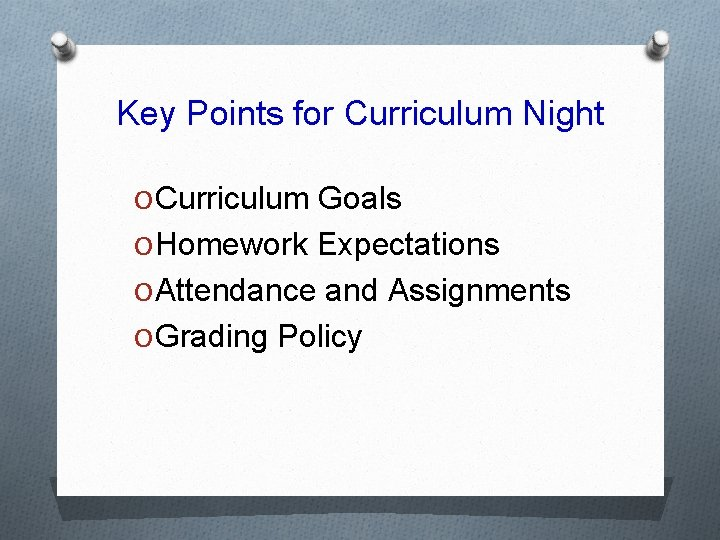Key Points for Curriculum Night O Curriculum Goals O Homework Expectations O Attendance and Assignments O Grading Policy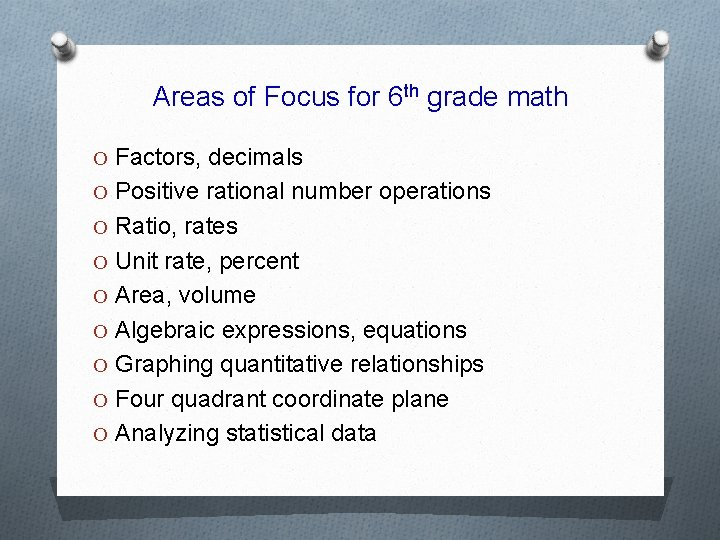Areas of Focus for 6 th grade math O Factors, decimals O Positive rational number operations O Ratio, rates O Unit rate, percent O Area, volume O Algebraic expressions, equations O Graphing quantitative relationships O Four quadrant coordinate plane O Analyzing statistical data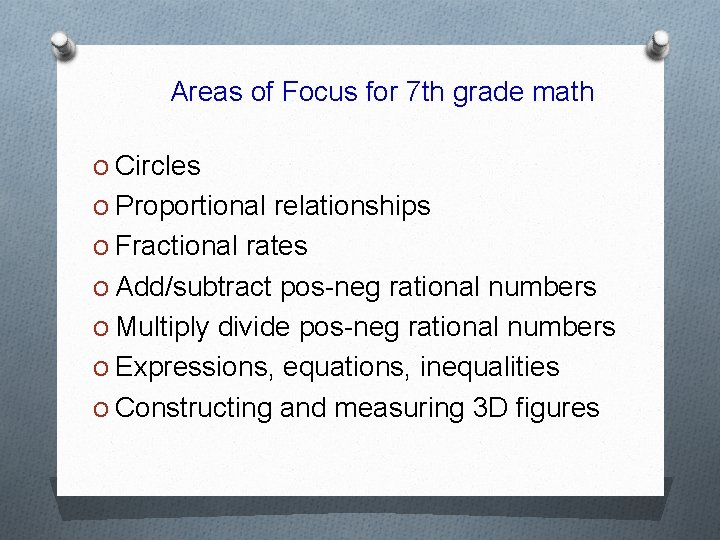Areas of Focus for 7 th grade math O Circles O Proportional relationships O Fractional rates O Add/subtract pos-neg rational numbers O Multiply divide pos-neg rational numbers O Expressions, equations, inequalities O Constructing and measuring 3 D figuresHomework O The purpose of homework is to practice, and/or reinforce concepts we are working on in class. Credit is given for completion, not perfection. O If your child has trouble with HW, he/she is expected to problem solve using any available resources (notes, textbook, websites, logic, reasoning). If they are unable to find a solution, they should come to class with any methods or strategies that were attempted and be prepared to ask questions. O Homework is assigned in class approximately 2 -4 nights per week, and will be posted on my website.Absences Homework and assessments must be made up when absent. Students have one day for every day absent to make up homework for full credit. When absent, it is the responsibility of student to: O Check Ms. Sunday’s website for class information, assignments and/or notes. O Copy class notes, complete and turn in missed assignments. O See teacher to schedule a convenient time to make-up missed assessments.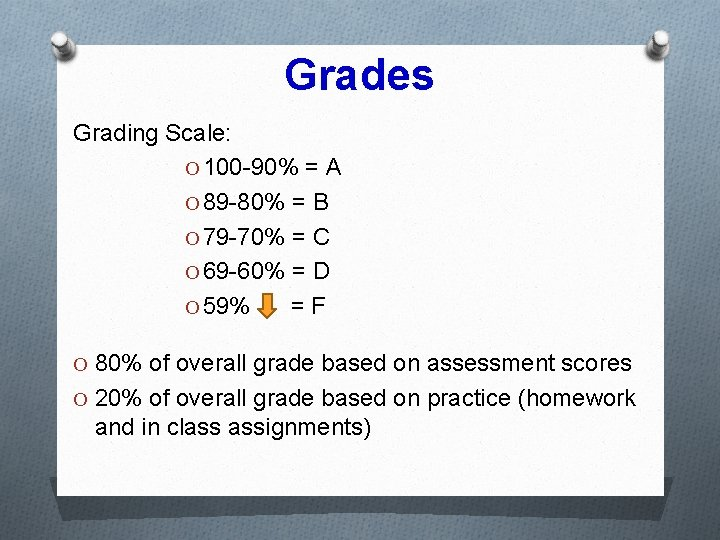Grades Grading Scale: O 100 -90% = A O 89 -80% = B O 79 -70% = C O 69 -60% = D O 59% =F O 80% of overall grade based on assessment scores O 20% of overall grade based on practice (homework and in class assignments)